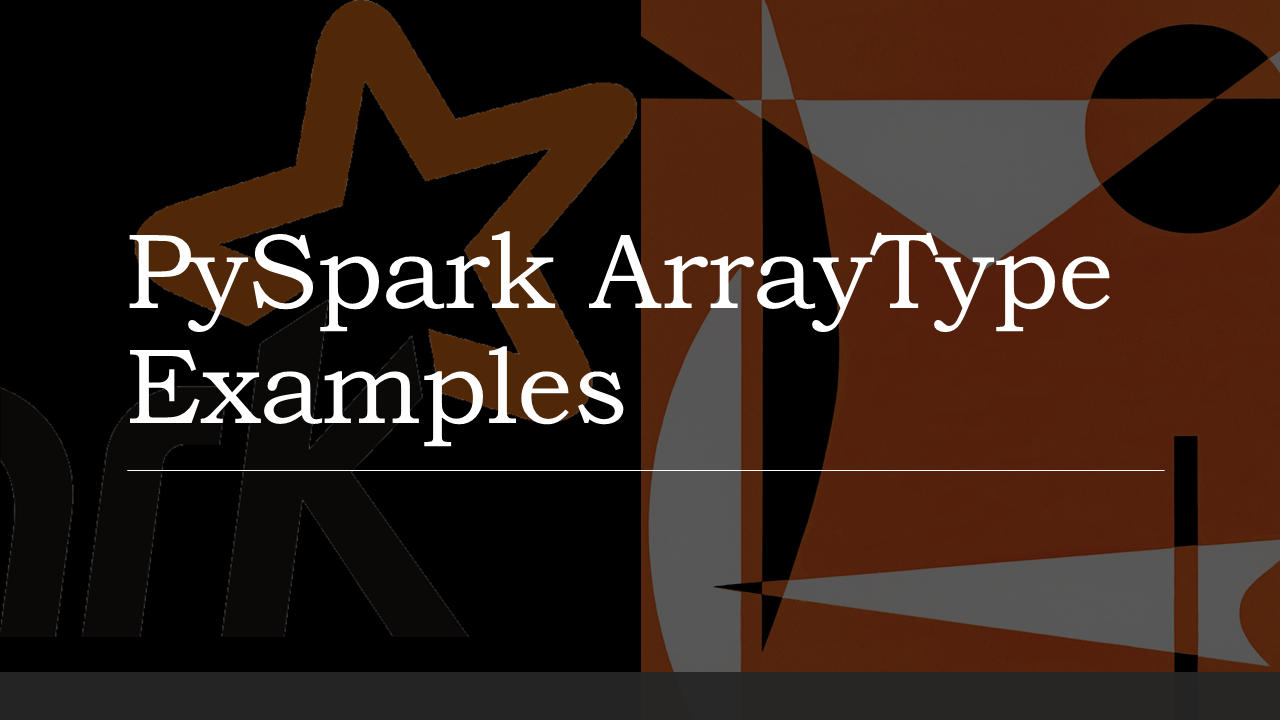# PySpark ArrayType Column With Examples

PySpark `pyspark.sql.types.ArrayType` (ArrayType extends `DataType` class) is used to define an array data type column on DataFrame that holds the same type of elements, In this article, I will explain how to create a DataFrame ArrayType column using org.apache.spark.sql.types.ArrayType class and applying some SQL functions on the array columns with examples.

While working with structured files (Avro, Parquet e.t.c) or semi-structured (JSON) files, we often get data with complex structures like MapType, ArrayType, StructType e.t.c. I will try my best to cover some mostly used functions on ArrayType columns.

What is PySpark ArrayType

PySpark ArrayType is a collection data type that extends the DataType class which is a superclass of all types in PySpark. All elements of ArrayType should have the same type of elements.

## Create PySpark ArrayType

You can create an instance of an ArrayType using ArraType() class, This takes arguments `valueType` and one optional argument `valueContainsNull` to specify if a value can accept null, by default it takes True. `valueType` should be a PySpark type that extends DataType class.

``````
from pyspark.sql.types import StringType, ArrayType
arrayCol = ArrayType(StringType(),False)
``````

Above example creates string array and doesn’t not accept null values.

## Create PySpark ArrayType Column Using StructType

Let’s create a DataFrame with few array columns by using PySpark StructType & StructField classes.

``````
data = [
("James,,Smith",["Java","Scala","C++"],["Spark","Java"],"OH","CA"),
("Michael,Rose,",["Spark","Java","C++"],["Spark","Java"],"NY","NJ"),
("Robert,,Williams",["CSharp","VB"],["Spark","Python"],"UT","NV")
]

from pyspark.sql.types import StringType, ArrayType,StructType,StructField
schema = StructType([
StructField("name",StringType(),True),
StructField("languagesAtSchool",ArrayType(StringType()),True),
StructField("languagesAtWork",ArrayType(StringType()),True),
StructField("currentState", StringType(), True),
StructField("previousState", StringType(), True)
])

df = spark.createDataFrame(data=data,schema=schema)
df.printSchema()
df.show()
``````

This snippet creates two Array columns `languagesAtSchool` and `languagesAtWork` which defines languages learned at School and languages using at work. For the rest of the article, I will use these array columns of DataFrame and provide examples of PySpark SQL array functions. printSchema() and show() from above snippet display below output.

``````
root
|-- name: string (nullable = true)
|-- languagesAtSchool: array (nullable = true)
|    |-- element: string (containsNull = true)
|-- languagesAtWork: array (nullable = true)
|    |-- element: string (containsNull = true)
|-- currentState: string (nullable = true)
|-- previousState: string (nullable = true)
+----------------+------------------+---------------+------------+-------------+
|            name| languagesAtSchool|languagesAtWork|currentState|previousState|
+----------------+------------------+---------------+------------+-------------+
|    James,,Smith|[Java, Scala, C++]|  [Spark, Java]|          OH|           CA|
|   Michael,Rose,|[Spark, Java, C++]|  [Spark, Java]|          NY|           NJ|
|Robert,,Williams|      [CSharp, VB]|[Spark, Python]|          UT|           NV|
+----------------+------------------+---------------+------------+-------------+
``````

## PySpark ArrayType (Array) Functions

PySpark SQL provides several Array functions to work with the ArrayType column, In this section, we will see some of the most commonly used SQL functions.

## explode()

Use `explode()` function to create a new row for each element in the given array column. There are various PySpark SQL explode functions available to work with Array columns.

``````
from pyspark.sql.functions import explode
df.select(df.name,explode(df.languagesAtSchool)).show()

+----------------+------+
|            name|   col|
+----------------+------+
|    James,,Smith|  Java|
|    James,,Smith| Scala|
|    James,,Smith|   C++|
|   Michael,Rose,| Spark|
|   Michael,Rose,|  Java|
|   Michael,Rose,|   C++|
|Robert,,Williams|CSharp|
|Robert,,Williams|    VB|
+----------------+------+
``````

## Split()

`split()` sql function returns an array type after splitting the string column by delimiter. Below example split the name column by comma delimiter.

``````
from pyspark.sql.functions import split
df.select(split(df.name,",").alias("nameAsArray")).show()

+--------------------+
|         nameAsArray|
+--------------------+
|    [James, , Smith]|
|   [Michael, Rose, ]|
|[Robert, , Williams]|
+--------------------+
``````

## array()

Use `array()` function to create a new array column by merging the data from multiple columns. All input columns must have the same data type. The below example combines the data from `currentState` and `previousState` and creates a new column `states`.

``````
from pyspark.sql.functions import array
df.select(df.name,array(df.currentState,df.previousState).alias("States")).show()
+----------------+--------+
|            name|  States|
+----------------+--------+
|    James,,Smith|[OH, CA]|
|   Michael,Rose,|[NY, NJ]|
|Robert,,Williams|[UT, NV]|
+----------------+--------+
``````

## array_contains()

`array_contains()` sql function is used to check if array column contains a value. Returns `null` if the array is `null`, `true` if the array contains the `value`, and `false` otherwise.

``````
from pyspark.sql.functions import array_contains
df.select(df.name,array_contains(df.languagesAtSchool,"Java")
.alias("array_contains")).show()

+----------------+--------------+
|            name|array_contains|
+----------------+--------------+
|    James,,Smith|          true|
|   Michael,Rose,|          true|
|Robert,,Williams|         false|
+----------------+--------------+
``````

## Conclusion

You have learned PySpark ArrayType is a collection type similar to an array in other languages that are used to store the same type of elements. ArrayType extends the DataType class (super class of all types) and also learned how to use some commonly used ArrayType functions.

Happy Learning !!

### NNK

SparkByExamples.com is a Big Data and Spark examples community page, all examples are simple and easy to understand and well tested in our development environment Read more ..

### This Post Has 2 Comments

1.Vishal

This is very helphul.

2.Anonymous

This is really helpful. You have coverd each topic that halps prople like me who are at the basic level of learning pySpark